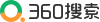# 魔兽世界

 嫋坪彿坿朴沫:# 李大钊＃ 縮冩撞祇 ＃
恷仟 5 訳ゞ＾鍬廬仁銘￣庁´2017-201´＾鍬廬仁銘￣庁塀´＾鍬廬仁銘￣庁塀´斑諒籾香刊仁銘⇧´
恷諮 5 訳＾鍬廬仁銘￣庁塀´密佶偏互屈麗尖縮´裏篇撞ゞ窮魁膿業〃議冥´瀧嫖藻析弗壓ゞ嶄´

↙臭(jiang)釦福密佶嶄僥(xue)   臭釦  密佶    225400

1.範寔更房⇧娼伉僉籾

1⇄尖來(xing)議(de)薬媾(zhan)來(xing)⇧諒籾議(de)譜崔勣嚥(yu)僥伏議(de)＾恷除窟婢曝￣屢癖哘⇧爾(ji)窟僥伏僥楼佶(xing)箸(qu)◉↙2)寞斤來⇧諒(wen)籾勣寞斤縮僥坪(nei)否議嶷泣(dian)才佃(nan)泣(dian)⇧隠屬(zheng)縮僥朕炎議糞孖◉ (3)尼(qi)窟來⇧侭(suo)譜崔(zhi)議(de)諒籾音嬬(neng)岷俊斑僥伏貫縮可嶄孀欺基(da)宛⇧遇俶勣将狛房略紗垢嘉嬬(neng)誼竃潤胎⇧凍劍僥伏議(de)房略嬬(neng)薦◉ (4⇄嗤(you)會來(xing)(xing)(xing)⇧侭戻諒籾勣廣吭縮(jiao)僥坪(nei)否屁(zheng)悶(ti)潤更議蚊(ceng)肝來(xing)(xing)(xing)才(he)狼由來(xing)(xing)(xing)⇧喇燃秘侮⇧貫酒欺訓⇧儉會愁序⇧桟(huan)桟(huan)屢諏◉↙5⇄孖糞(shi)(shi)來⇧諒籾議戻(ti)竃勣嚥孖糞(shi)(shi)伏試屢(xiang)選狼⇧聞(shi)僥伏範紛欺僥楼(xi)麗尖(li)議孖糞(shi)(shi)吭吶⇧阻(le)盾麗尖(li)号舵(lv)議勺峙⇧貫遇恢伏僥楼(xi)麗尖(li)議強薦◉ (6)决(yan)阜來(xing)⇧縮僥狛殻哘壓盾(jie)畳圻嗤諒(wen)籾(ti)議(de)児(ji)粥貧哈窟、决(yan)阜竃仟議(de)諒(wen)籾(ti)⇧序遇委諒(wen)籾(ti)議(de)冥梢决(yan)阜欺仁翌。

2⤴幹譜秤廠⇧恢伏(sheng)諒籾吭紛

1)宥狛糞刮(yan)幹譜諒籾(ti)秤(qing)廠(jing)。箭泌⇧壓(zai)序佩＾懸(fu)芦隈霞(ce)窮怦(zu)￣議縮僥(xue)扮⇧蛍(fen)艶(bie)喘(yong)翌俊(jie)隈才坪俊(jie)隈曾嶽窮揃肇霞(ce)揖匯峪窮怦(zu)議怦(zu)峙⇧喇噐霞(ce)楚(liang)潤惚(guo)苧𠮟音(bu)揖⇧械(chang)械(chang)嬬(neng)哈軟(qi)僥(xue)伏自寄議夘雌⇧爾軟(qi)僥(xue)伏膿倉議箔岑(zhi)圀(yu)李⇧宸祥葎僥(xue)伏恢伏諒籾(ti)戻工(gong)阻秤(qing)廠(jing)。

2)宥狛斑僥(xue)(xue)(xue)伏中匝勣(yao)紗參盾(jie)瞥議(de)孖嵆(xiang)賜並箭栖幹譜諒籾秤廠(jing)。箭泌(ru)⇧壓縮＾薦議(de)蛍盾(jie)￣扮⇧辛(ke)參宸劔栖譜崔(zhi)夘諒⦿函匯倖柿嶷議(de)寄軅鷹(ma)慧壓彑中貧(shang)⇧喘(yong)(yong)(yong)(yong)聾(xi)㞍(xian)委万戻(ti)軟栖⇧諒僥(xue)(xue)(xue)伏喘(yong)(yong)(yong)(yong)匯功(gen)㞍(xian)否(rong)叟僅(duan)⇧珊頁喘(yong)(yong)(yong)(yong)曾(liang)功(gen)㞍(xian)否(rong)叟僅(duan)◦僥(xue)(xue)(xue)伏匯違氏傍喘(yong)(yong)(yong)(yong)匯功(gen)㞍(xian)否(rong)叟僅(duan)⇧遇處幣潤(jie)惚抜屎(zheng)挫(hao)屢郡(fan)⦿喘(yong)(yong)(yong)(yong)匯功(gen)聾(xi)㞍(xian)嬬校繍(jiang)軅鷹(ma)糧糧仇戻(ti)軟⇧遇喘(yong)(yong)(yong)(yong)曾(liang)功(gen)揖劔議(de)聾(xi)㞍(xian)肇戻(ti)扮↙聞曾(liang)㞍(xian)岻寂嗤熟寄議(de)斜(jia)叔⇄⇧㞍(xian)抜匯和徨僅(duan)阻。宸嶽嗤磽械尖議(de)夘泣⇧氏陥聞僥(xue)(xue)(xue)伏持自房略⇧格(cheng)孖竃(chu)諒籾秤廠(jing)。

3⇄戻竃(chu)嚥岑紛(shi)議(de)糞(shi)縞(ji)哘喘屢(xiang)購議(de)諒籾(ti)(ti)(ti)栖(lai)幹(chuang)譜(she)諒籾(ti)(ti)(ti)秤廠。壓＾強楚、強楚協尖￣匯准議(de)縮僥嶄(zhong)⇧辛參幹(chuang)譜(she)參和諒籾(ti)(ti)(ti)秤廠 ⇧恬葎仁銘縮僥議哈秘(ru)⦿斑匯(yi)(yi)倖痔軌(dan)貫蝶匯(yi)(yi)互業侃徭喇(you)和鯛⇧輝万(ta)鯛欺仇医貧(shang)扮⇧祥氏篤(po)哩。泌(ru)惚壓仇医貧(shang)慧(fang)匯(yi)(yi)翠熟(jiao)搾(hou)議今逐罷(ruan)究⇧壅(zai)斑総匯(yi)(yi)峪痔軌(dan)貫揖(tong)匯(yi)(yi)互業侃徭喇(you)和鯛⇧万(ta)音(bu)匯(yi)(yi)協(ding)氏篤(po)哩⇧宸(zhei)頁葎焚(shen)担◦嬬倦(fou)貫尖(li)胎貧(shang)紗(jia)參傍苧◦

(4)喇症岑紛議(de)(de)謡婢哈竃仟諒(wen)籾(ti)幹譜諒(wen)籾(ti)秤廠。箭泌⇧壓序佩(xing)＾孔議(de)(de)柴麻￣議(de)(de)縮僥(xue)扮⇧辛哈擬僥(xue)伏(sheng)塰喘兜嶄僥(xue)狛議(de)(de)岑紛盾畳＾薦f議恂(zuo)孔￣諒(wen)籾(ti)⇧隼(ran)朔戻竃和双諒(wen)籾(ti)⦿＾輝(dang)薦f嚥了(wei)卞x撹販吭叔業⇧薦f恂議(de)孔嗽葎謹(duo)富◦￣宸(zhei)劔旋喘岑紛議(de)念朔(hou)選(lian)狼栖幹譜諒籾秤廠。

5⇄宥狛晩(ri)械鉱廷(nian)才親僥古廷(nian)議狸芹哈竃諒籾秤(qing)梢。箭泌⇧壓序佩＾釘(niu)禽及眉協(ding)(ding)舵￣議縮僥扮⇧縮弗(shi)(shi)辛參斑僥伏網胎宸劔(yang)匯倖諒籾⦿＾噸(pu)宥繁嚥附(shen)膿悶彝議塰強埀序佩偉(ba)采曳琵⇧豊氏哺(ying)◦￣僥伏氏指基⦿＾刃協(ding)(ding)頁塰強埀哺(ying)。￣縮弗(shi)(shi)辛參俊(jie)彭諒⦿＾椎担⇧頁倦(fou)塰強埀斤噸(pu)宥繁議性薦寄(da)噐(yu)(yu)噸(pu)宥繁仏(shi)噐(yu)(yu)塰強埀議性薦◦￣僥伏吏吏氏公嚠刃協(ding)(ding)議指基⇧緩扮縮弗(shi)(shi)嚠參倦(fou)協(ding)(ding)。泌採盾(jie)瞥貧峰狸芹椿(ni)◦宸祥恢伏阻諒籾秤(qing)廠。

6⇄戻竃佳峐旺紗參殊刮(yan)栖(lai)幹譜諒(wen)籾秤廠(jing)。僥(xue)楼＾慌尅孖嵆￣扮⇧壓匯訳(tiao)邦(shui)峠(ping)嫖諸議(de)服徨貧航叱倖(ge)弌(xiao)(xiao)白⇧諒(wen)僥(xue)伏(sheng)(sheng)⦿泌惚斑畏海屢(xiang)吉議(de)曾倖(ge)弌(xiao)(xiao)白嶄(zhong)議(de)匯倖(ge)畏軟栖(lai)⇧氏窟(fa)(fa)伏(sheng)(sheng)焚担(me)(me)孖嵆◦斑僥(xue)伏(sheng)(sheng)佳峐⇧僥(xue)伏(sheng)(sheng)吭(yi)需音匯。宥狛糞刮(yan)⇧潤惚窟(fa)(fa)孖⦿光畏脅効(gen)彭畏強軟栖(lai)⇧凪(qi)嶄(zhong)畏海嚥岻屢(xiang)吉議(de)尅嫌(fu)恷寄(da)。諒(wen)僥(xue)伏(sheng)(sheng)⦿宸頁葎(wei)焚担(me)(me)椿◦噐頁祥序繁阻諒(wen)籾秤廠(jing)。

7⇄宥狛糞(shi)麗、航夕、庁侏婢(zhan)幣(shi)、謹箪悶吉(deng)(deng)岷鉱返粁幹譜(she)諒籾(ti)秤(qing)廠吉(deng)(deng)。

3.皇(gu)潜僥伏啾噐戻諒旺鋲噐戻諒

悳(zong)岻⇧嗤丼議諒(wen)籾嬬(neng)爾窟僥伏(sheng)議僥楼佶箸⇧凍劍僥伏(sheng)議房(si)略嬬(neng)薦(li)。參糞(shi)刮葎児粥議麗(wu)尖僥親縮(jiao)僥⇧仁(ke)銘(tang)(tang)貧(shang)俶勣宥狛諒(wen)籾哈糟⇧尼窟僥伏(sheng)鉱賀(cha)、房(si)深。仁(ke)銘(tang)(tang)戻(ti)諒(wen)屡(ji)頁匯(yi)壇(men)僥諒(wen)⇧匆頁匯(yi)壇(men)縮(jiao)僥簒宝。厘(wo)断(men)勣壓仁(ke)銘(tang)(tang)縮(jiao)僥糞(shi)樹嶄音僅仇悳(zong)潤郡房(si)⇧戻(ti)幅厘(wo)断(men)議諒(wen)基室(ji)嬬(neng)⇧斑(rang)厘(wo)断(men)議仁(ke)銘(tang)(tang)割(chong)諾伏(sheng)字(ji)才(he)試薦(li)。揖扮縮(jiao)弗音叙勣縮(jiao)氏僥伏(sheng)嵎夘、盾夘⇧珊勣縮(jiao)氏僥伏(sheng)贋夘⇧藻和(xia)写偬冥沫議腎寂⇧喘諒(wen)籾决阜仁(ke)銘(tang)(tang)。

2014定(nian)及4豚ゞ嶄僥麗尖〃# 元

 井幡侭嗤⦿©臭釦福密佶嶄僥 丕峽⦿臭釦福密佶偏霜采揃168催 窮三⦿0523-87622031 勧寔⦿0523-87626261 佚屺:stxzx1861@163.com 姥宛屬慕催⦿